# PH8151 Notes Engineering Physics Regulation 2017 Anna University

PH8151 Notes Engineering Physics Regulation 2017 Anna University

## OBJECTIVES: ph8151 notes engineering physics

 To enhance the fundamental knowledge in Physics and its applications relevant to various streams of Engineering and Technology.

### OUTCOMES: ph8151 notes engineering physics

Upon completion of this course,

 the students will gain knowledge on the basics of properties of matter and its applications,

 the students will acquire knowledge on the concepts of waves and optical devices and their applications in fibre optics,

 the students will have adequate knowledge on the concepts of thermal properties of materials and their applications in expansion joints and heat exchangers,

 the students will get knowledge on advanced physics concepts of quantum theory and its applications in tunneling microscopes, and

 the students will understand the basics of crystals, their structures and different crystal growth techniques.

#### TEXT BOOKS: ph8151 notes engineering physics

1. Bhattacharya, D.K. & Poonam, T. “Engineering Physics”. Oxford University Press, 2015.

2. Gaur, R.K. & Gupta, S.L. “Engineering Physics”. Dhanpat Rai Publishers, 2012.

3. Pandey, B.K. & Chaturvedi, S. “Engineering Physics”. Cengage Learning India, 2012.

##### REFERENCES: ph8151 notes engineering physics

1. Halliday, D., Resnick, R. & Walker, J. “Principles of Physics”. Wiley, 2015.

2. Serway, R.A. & Jewett, J.W. “Physics for Scientists and Engineers”. Cengage Learning, 2010.

3. Tipler, P.A. & Mosca, G. “Physics for Scientists and Engineers with Modern Physics’. W.H.Freeman, 2007.

###### Padeepz E-Learning Materials PH8151 Engineering Physics

Padeepz E-Learning Materials PH8151 Engineering Physics we have provided the sample materials in this page.  If you like the sample and want to buy the full subject the procedure is also provided in this page.

###### Modulus of elasticity

Types of moduli of elasticity:

Based on the 3 types of strain, elastic modulli of solids are.

(i) young’s Modulus:

Within elastic limit, ratio of longitudinal stress to longitudinal strain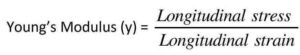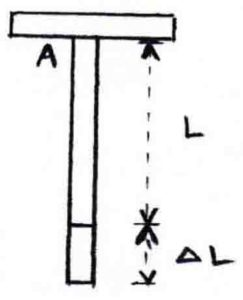consider a wire, length – L

Area of cross section – a

One end is fixed at top and load is applied at the bottom. Force acting along the length of wire is `F’. Let  – elongation in length of the wire due to force applied.

Longitudinal stress = F/A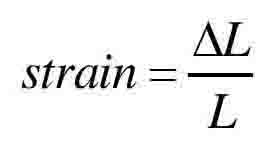(II) Bulk Modulus:

Within elastic limit, ratio of bulk stress to bulk stress.

Bulk Modulus (k) = Bulk stress / Bulk Strain

Consider a body of volume ‘v’ and area of cross section A let ‘F’ the force applied to the whole surface of the body. This results in the change in volume but no change in shape of the body. Let v he the change in volume.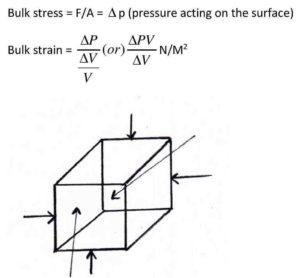Bulk modules is sometimes called in compressibility its reciprocal is compressibility =1/k

(iii)Rigidity Modulus

Within elastic limit, ratio of sharing stress to shearing strain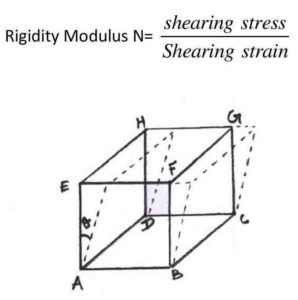Consider cube ABCDEFGH . lower ABCD is fixed and force is applied 11l to surface EFGH. So the body is sheared by angle o . Let ‘L’ he the length of the cube and sL be the change in length of the upper surface

Shearing stress = F/A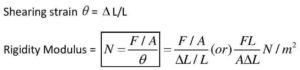Poisson’s Ratio:

When a body is subjected to a force, the deformation is not only in one direction but all along.

Ex: If a wire is stretched, besides undergo extension along the direction of force, it also undergoes contraction in perpendicular direction strain produced along the direction of force longitudinal strain

Similarly, strain produced in perpendicular direction is lateral strain

Within elastic limit, ratio, of lateral strain to the longitudinal strain is called poisson’s ratio ‘’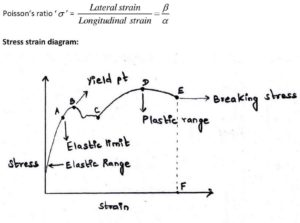Let us consider a body which is subjected to an uniformly increasing stress Due to the application of stress, strain is developed If we plot a graph b/w stress & strain we get a curve and is called stress. Strain diagram

1. Itis found that the bodyobey’s Hooke’s law up to the region OA called elastic lang
2. As soon as the yieldpt‘B’ is crossed, the strain & rapidly than the stress
3. Atthis stage the body remains partly elastic and partly plastic which is represented by BC
4. Noweven if a small external face is applied, the body will take a new path CD and remains plastic called plastic law where D is the ultimate strength.
5. Afterthis, the body will not some to its Original state and the body acquires a permanent residual strain I and it breaks down at aptl/ called a breaking stress indicated by EF.
 Subject name Engineering Physics Subject Code PH8151 Semester 1 Regulation 2017 Regulation

PH8151 Syllabus Engineering Physics

PH8151 Important questions Engineering Physics

PH8151 Question Bank Engineering Physics

## One Reply to “PH8151 Notes Engineering Physics Regulation 2017 Anna University”

1.Thangaraj says: